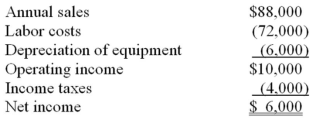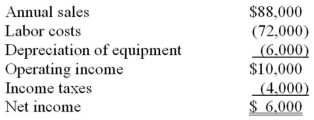• FAQ
• Contact/ Homework Answers / Accounting / 41.The principal weakness of the payback method for evaluating proposed
Not my Question
Flag Content

# Question : 41.The principal weakness of the payback method for evaluating proposed

41.The principal weakness of the payback method for evaluating proposed investments is that it does not:

A. provide a way of ranking projects in order of desirability.

B. consider cash flows that continue after the investment has been recovered.

C. result in an easily understood "answer".

D. recognize the time value of money.

42.The accounting rate of return method for evaluating proposed investments:

A. is based on cash receipts and disbursements related to the investment.

B. uses accounting net income from the operating budget.

C. does not recognize the time value of money.

D. is easier to use than the net present value method.

43.The capital budgeting analytical technique that calculates the rate of return on the investment based on the impact of the investment on the financial statements is known as the:

A. internal rate of return.

B. accounting rate of return.

C. payback period.

D. net present value.

44.An advantage of the net present value method for evaluating investment proposals over the internal rate of return method is that:

A. only one set of present value calculations using a required discount rate is made.

B. the actual rate of return on the project is calculated.

C. projects can be ranked in order of profitability using the net present value amount.

D. estimates of future cash flows do not have to be made.

45.If an asset costs \$16,000, has an expected useful life of 8 years, is expected to have a \$2,000 salvage value and generates net annual cash inflows of \$2,000 a year, the cash payback period is

A. 8 years.

B. 7 years.

C. 6 years.

D. 5 years.

46.Which of the following statements are true regarding the payback period?

A. The time value of money is considered when calculating the payback.

B. The payback analysis is more accurate than the net present value analysis.

C. The payback period is less accurate than the accounting rate of return.

D. The time value of money is not considered when calculating the payback.

47.Boccardi Inc., has invested in new pasta manufacturing equipment at a cost of \$48,000. The equipment has an estimated useful life of eight years. Estimated annual sales and operating expenses related to the pasta equipment follow:The estimated payback of the investment in the pasta equipment is:

A. 3.0 years.

B. 4.0 years.

C. 6.0 years.

D. 8.0 years.

48.Boccardi Inc., has invested in new pasta manufacturing equipment at a cost of \$48,000. The equipment has an estimated useful life of eight years. Estimated annual sales and operating expenses related to the pasta equipment follow:The estimated accounting rate of return is:

A. 12.5%.

B. 18.0%.

C. 25.0%.

D. 33.3%.

49.In a capital budgeting decision, if a firm uses the net present value method and a 12% discount rate, what does a negative net present value indicate?

A. The proposal's rate of return exceeds 12%.

B. The proposal's rate of return is less than the minimum rate required.

C. The proposal earns a rate of return between 10% and 12%.

D. None of these.

50.A capital budgeting decision method that considers the time value of money is the

A. accounting rate of return method.

B. return on stockholders' equity method.

C. cash payback method.

D. internal rate of return method.

16-1

## Solution 5 (1 Ratings )

Solved
Accounting 5 Months Ago 552 Views